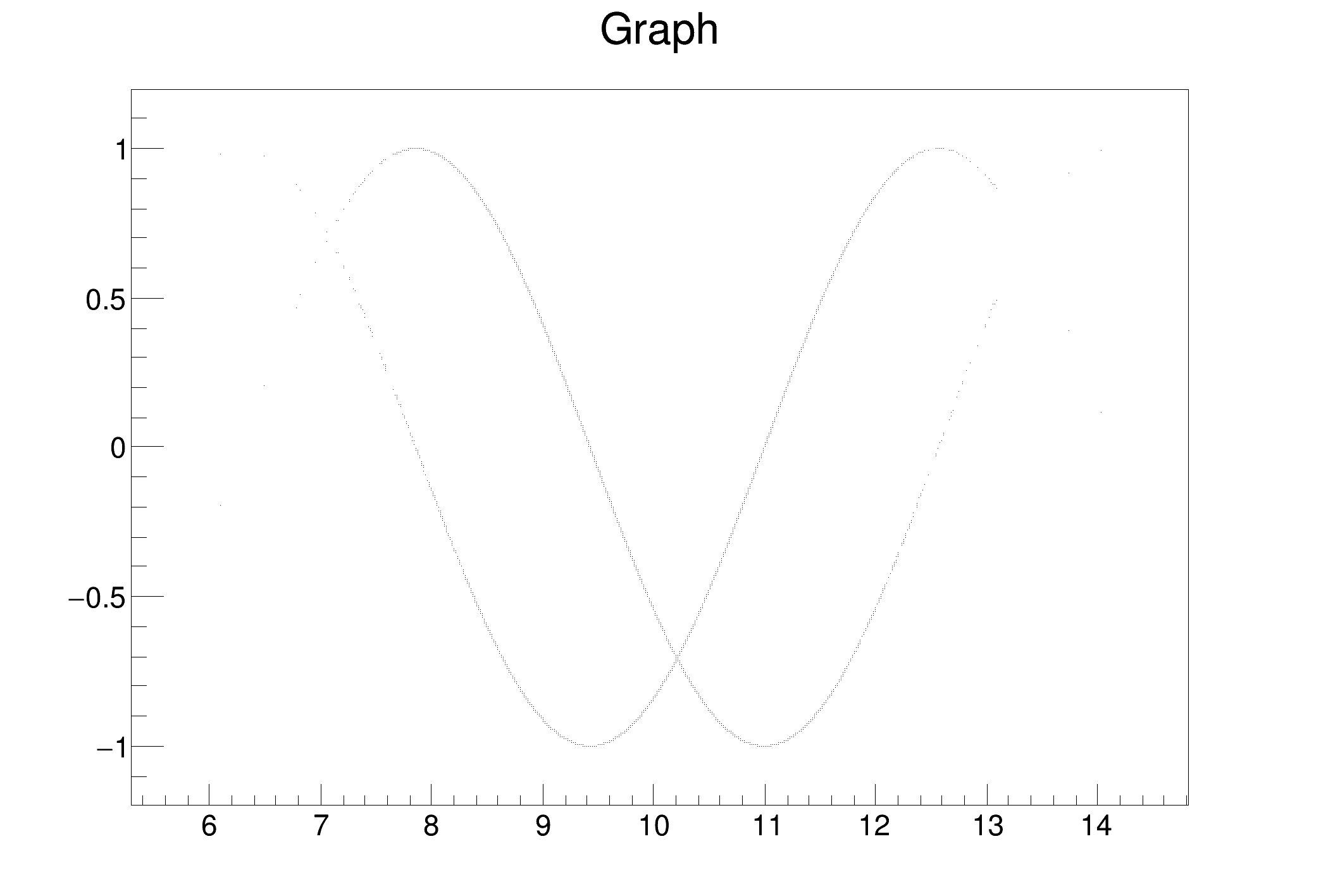ROOT   Reference Guide
Searching...
No Matches
treegetval.C File Reference

## Detailed DescriptionIllustrates how to retrieve TTree variables in arrays.

This example:

• creates a simple TTree,
• generates TTree variables thanks to the Draw method with goff option,
• retrieves some of them in arrays thanks to GetVal,
• generates and draw graphs with these arrays.

The option goff in TTree::Draw behaves like any other drawing option except that, at the end, no graphics is produced ( goff= graphics off). This allows to generate as many TTree variables as needed. All the graphics options (except para and candle) are limited to four variables only. And para and candle need at least two variables.

Note that by default TTree::Draw creates the arrays obtained with GetVal with a length corresponding to the parameter fEstimate. By default fEstimate=1000000 and can be modified via TTree::SetEstimate. To keep in memory all the results use:

tree->SetEstimate(-1);
Definition tree.py:1

SetEstimate should be called if the expected number of selected rows is greater than 1000000.The arrays' dimension is 5000
void treegetval() {
// create a simple TTree with 5 branches
Int_t run, evt;
Float_t x,y,z;
TTree *T = new TTree("T","test friend trees");
T->Branch("Run",&run,"Run/I");
T->Branch("Event",&evt,"Event/I");
T->Branch("x",&x,"x/F");
T->Branch("y",&y,"y/F");
T->Branch("z",&z,"z/F");
for (Int_t i=0;i<10000;i++) {
if (i < 5000) run = 1;
else run = 2;
evt = i;
x = r.Gaus(10,1);
y = r.Gaus(20,2);
z = r.Landau(2,1);
T->Fill();
}
// Draw with option goff and generate seven variables
Int_t n = T->Draw("x:y:z:Run:Event:sin(x):cos(x)","Run==1","goff");
printf("The arrays' dimension is %d\n",n);
// Retrieve variables 0, 5 et 6
Double_t *vx = T->GetVal(0);
Double_t *vxs = T->GetVal(5);
Double_t *vxc = T->GetVal(6);
// Create and draw graphs
TGraph *gs = new TGraph(n,vx,vxs);
TGraph *gc = new TGraph(n,vx,vxc);
gs->Draw("ap");
gc->Draw("p");
}
ROOT::R::TRInterface & r
Definition Object.C:4
int Int_t
Definition RtypesCore.h:45
double Double_t
Definition RtypesCore.h:59
float Float_t
Definition RtypesCore.h:57
A TGraph is an object made of two arrays X and Y with npoints each.
Definition TGraph.h:41
virtual void Draw(Option_t *chopt="")
Draw this graph with its current attributes.
Definition TGraph.cxx:769
This is the base class for the ROOT Random number generators.
Definition TRandom.h:27
A TTree represents a columnar dataset.
Definition TTree.h:79
Double_t y[n]
Definition legend1.C:17
Double_t x[n]
Definition legend1.C:17
const Int_t n
Definition legend1.C:16

Definition in file treegetval.C.